RICalcFinancialInvestmentInflationGraphingStatisticsScientific / Other DownloadsFree demo Online shopBuy online AboutContact us LinksResources# Graphing calculatorRICalc is an essential Graphic calculator for maths.

You will find the Graphing calculator invaluable whether your are at high-school, at university or regularly deal with mathematics.

It is comprehensive, advanced and easy to use.

With RICalc you concentrate on the maths, not on the calculator. It is fun and simple to use with no cumbersome and complicated buttons.

General calculus, algebra, derivatives, integrals and function plotting is simple in RICalc.

With the Graphic calculator you have all the essential tools to solve and graph equations.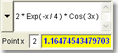• Solve an equation at point x.
Enter a one-dimensional algebraic equation and solve it for a given value.
Retrieve previous equation from a list of the last 100.• Use any of the 400+ pre-defined functions:
Math, Trigonometry, Statistic, Financial, Conversion, Logical, Text, Date, Geometry and Miscellaneous functions. Use your own saved expressions.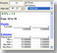• Get a Report on an equation for a given interval.
Type an algebraic equation, define point a and point b, and get a summary for:
Root(s), Extrema, Intercept, Distance and Table points.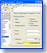Solver
• Solve equations with Root Finder numeric methods.
Find the root of an equation with choice of numeric methods:
Brent, Ridders, Newton-Raphson, Bisection, False position, Secant ...
Find the Extrema, the Minimum or the Maximum of a function.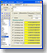Derivative
• Calculate Derivatives with choice of numeric differentiation methods.
Stirling, Newton, Ridders central, 3-5 points, forward, backward,
1st, 2nd, 3rd ... Nth derivative ... + 3 point high-precision derivative.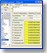Integral
• Calculate Integrals with choice of numeric integration methods.
Romberg (adaptive), Guass 3 & 5 points, Simpson 3/8 & 1/3,
Trapezoidal, Mid point, Rectangular Left & RightPoints
• Manually define matrix table points.
Define and type the table matrix points on a spreadsheet rows and columns.
Smooth adjust curves with low, medium and high interpolation.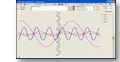Plot
• Plot equation(s) in 2D Rectangular, Parametric and Polar coordinates.
. Graph multiple equations.
. Mix rectangular, parametric or polar representations.
. Plot Tangent or Normal at a point.
. Plot Distance between points.
. Plot Derivative.
. Trace expression on the plane with mouse or use keyboard for fine steps.
. Zoom all or individual x and y, move up, down, left, right, centre or restore.
. Control plot Steps and Interval, Limits, Points or Lines
. Control plot Maximum and Minimum, style, width, colour ...Examples

RICalc not just software, also an educational investment tool.Graphing calculator

All-in-one true calculator, not several, just one.

Graphing, Scientific , Statistics , Inflation , Financial , Investment® Product and company names are trademarks or registered trademarksPrivacyDisclaimerSite Map © 2020 DBCOM software